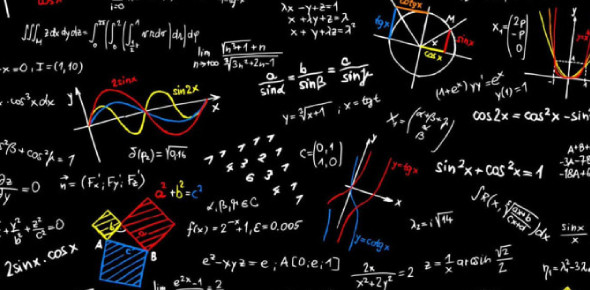# Everything From Algebra To Physics! Only The Smartest Can Crack This Test.

10 Questions | Attempts: 90SettingsPhysics is the natural science that studies matter, its motion and behavior through space and time, and the related entities of energy and force. Physics is one of the most fundamental scientific disciplines. Whereas, Mathematics includes the study of such topics as quantity, structure, space, and change. It has no generally accepted definition This Quiz Has Everything From Algebra To Physics! Only The Smartest Can Crack This Test.

• 1.
If u= 2i-j+3k. The magnitude of its vector product with itself is:
• A.

√14

• B.

14

• C.

12

• D.

0

• 2.
3x2+2√x+7=0 is a polynomial over:
• A.

Z

• B.

R

• C.

N

• D.

W

• 3.
Inverse of a Column Matrix is:
• A.

Row Matrix

• B.

Column Matrix

• C.

Symmetric

• D.

None of these

• 4.
An infinite geometric sequence containing all the numbers from the set of R can’t be a/an:
• A.

Fluctuating Sequence

• B.

Oscillatory Sequence

• C.

Constant Sequence

• D.

Geometric Sequence

• 5.
If 7th term of an A.P is 24 & 12th term is 49. Find the 15th term:
• A.

74

• B.

66

• C.

64

• D.

56

• 6.
The smaller a physical quantity to be measured, the more _____ instrument should be used:
• A.

Accurate

• B.

Precise

• C.

Both

• D.

Depends on the quantity

• 7.
Which color is not used in color printing?
• A.

Magenta

• B.

Cyan

• C.

Black

• D.

Green

• 8.
Rate of change of momentum if a force of 2N acts on a body for 4s is:
• A.

4

• B.

8

• C.

2

• D.

0.5

• 9.
Time taken by light to reach Earth from the Moon is:
• A.

8 min 20s

• B.

5 min 20s

• C.

1 min 20s

• D.

5 min 10s

• 10.
Area of a room having length 2m & width 5m with absolute uncertainties 1m & 2.5m respectively in m2 might possibly be:
• A.

0, 20

• B.

9, 11

• C.

0, 10

• D.

10, 20

## Related TopicsBack to top
×

Wait!
Here's an interesting quiz for you.